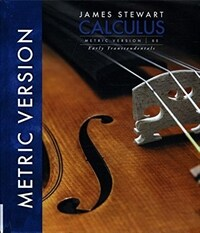### Calculus: Early Transcendentals (Hardcover, 8th International)

ISBN
9781305272378
ISBN(10)
1305272374
저자
James Stewart
출판사
Cengage Learning
출판일
2015.09.30
형태
1,368페이지 / 미확인
태그
Cengage_Learning,James_Stewart,외국도서,자연과학,수학,수리분석
평점

0점

0%

정가 45,000

할인가 45,000

#### 목차

Preface. To the Student. Diagnostic Tests. A Preview of Calculus. 1. FUNCTIONS AND MODELS. Four Ways to Represent a Function. Mathematical Models: A Catalog of Essential Functions. New Functions from Old Functions. Exponential Functions. Inverse Functions and Logarithms. Review. Principles of Problem Solving. 2. LIMITS AND DERIVATIVES. The Tangent and Velocity Problems.The Limit of a Function.Calculating Limits Using the Limit Laws. The Precise Definition of a Limit. Continuity. Limits at Infinity; Horizontal Asymptotes. Derivatives and Rates of Change. Writing Project: Early Methods for Finding Tangents.The Derivative as a Function. Review. Problems Plus. 3. DIFFERENTIATION RULES. Derivatives of Polynomials and Exponential Functions. Applied Project: Building a Better Roller Coaster. The Product and Quotient Rules. Derivatives of Trigonometric Functions. The Chain Rule. Applied Project: Where Should a Pilot Start Descent? Implicit Differentiation. Laboratory Project: Families of Implicit Curves. Derivatives of Logarithmic Functions. Rates of Change in the Natural and Social Sciences. Exponential Growth and Decay. Applied Project: Controlling Red Blood Cell Loss During Surgery. Related Rates. Linear Approximations and Differentials. Laboratory Project: Taylor Polynomials. Hyperbolic Functions. Review. Problems Plus. 4. APPLICATIONS OF DIFFERENTIATION. Maximum and Minimum Values. Applied Project: The Calculus of Rainbows. The Mean Value Theorem. How Derivatives Affect the Shape of a Graph. Indeterminate Forms and l'Hospital's Rule. Writing Project: The Origins of l'Hospital's Rule. Summary of Curve Sketching. Graphing with Calculus and Calculators. Optimization Problems. Applied Project: The Shape of a Can. Applied Project: Planes and Birds: Minimizing Energy. Newton's Method. Antiderivatives. Review. Problems Plus. 5. INTEGRALS. Areas and Distances. The Definite Integral. Discovery Project: Area Functions. The Fundamental Theorem of Calculus. Indefinite Integrals and the Net Change Theorem. Writing Project: Newton, Leibniz, and the Invention of Calculus. The Substitution Rule. Review. Problems Plus. 6. APPLICATIONS OF INTEGRATION. Areas Between Curves. Applied Project: The Gini Index. Volume. Volumes by Cylindrical Shells. Work. Average Value of a Function. Applied Project: Calculus and Baseball. Applied Project: Where to Sit at the Movies. Review. Problems Plus. 7. TECHNIQUES OF INTEGRATION. Integration by Parts. Trigonometric Integrals. Trigonometric Substitution. Integration of Rational Functions by Partial Fractions. Strategy for Integration. Integration Using Tables and Computer Algebra Systems. Discovery Project: Patterns in Integrals. Approximate Integration. Improper Integrals. Review. Problems Plus. 8. FURTHER APPLICATIONS OF INTEGRATION. Arc Length. Discovery Project: Arc Length Contest. Area of a Surface of Revolution. Discovery Project: Rotating on a Slant. Applications to Physics and Engineering. Discovery Project: Complementary Coffee Cups. Applications to Economics and Biology. Probability. Review. Problems Plus. 9. DIFFERENTIAL EQUATIONS. Modeling with Differential Equations. Direction Fields and Euler's Method. Separable Equations. Applied Project: How Fast Does a Tank Drain? Applied Project: Which is Faster, Going Up or Coming Down? Models for Population Growth. Linear Equations. Predator-Prey Systems. Review. Problems Plus. 10. PARAMETRIC EQUATIONS AND POLAR COORDINATES. Curves Defined by Parametric Equations. Laboratory Project: Families of Hypocycloids. Calculus with Parametric Curves. Laboratory Project: Bezier Curves. Polar Coordinates. Laboratory Project: Families of Polar Curves. Areas and Lengths in Polar Coordinates. Conic Sections. Conic Sections in Polar Coordinates. Review. Problems Plus. 11. INFINITE SEQUENCES AND SERIES. Sequences. Laboratory Project: Logistic Sequences. Series. The Integral Test and Estimates of Sums. The Comparison Tests. Alternating Series. Absolute